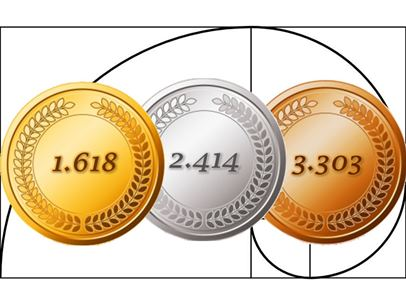# Activities

•• ##### AuthorAust Senior

30 Minutes

• ##### Device
•TI-84 Plus CE

## Metallic Numbers#### Activity Overview

The Golden Ratio and its association with the Fibonacci sequence is well know, but there is much more to explore. Variants of the Fibonacci sequence of the form t(n+2)=t(n)+a.t(n+1) also generate specific ratios. For a = 2 the ratio is referred to as the Silver Ratio, for a = 3, the Bronze Ratio. Collectively these are called the metallic ratios where a = 1 is the specific case and equals the Golden Ratio.

#### Objectives

• Use recursion to generate a sequence
• Solve problems involving sequences
• Explore the most popular second order linear recursive relation

#### Vocabulary

• Recursion
• Fibonacci Sequence
• Golden Ratio
• Silver Ratio
• Bronze Ratio
• Metallic Ratios